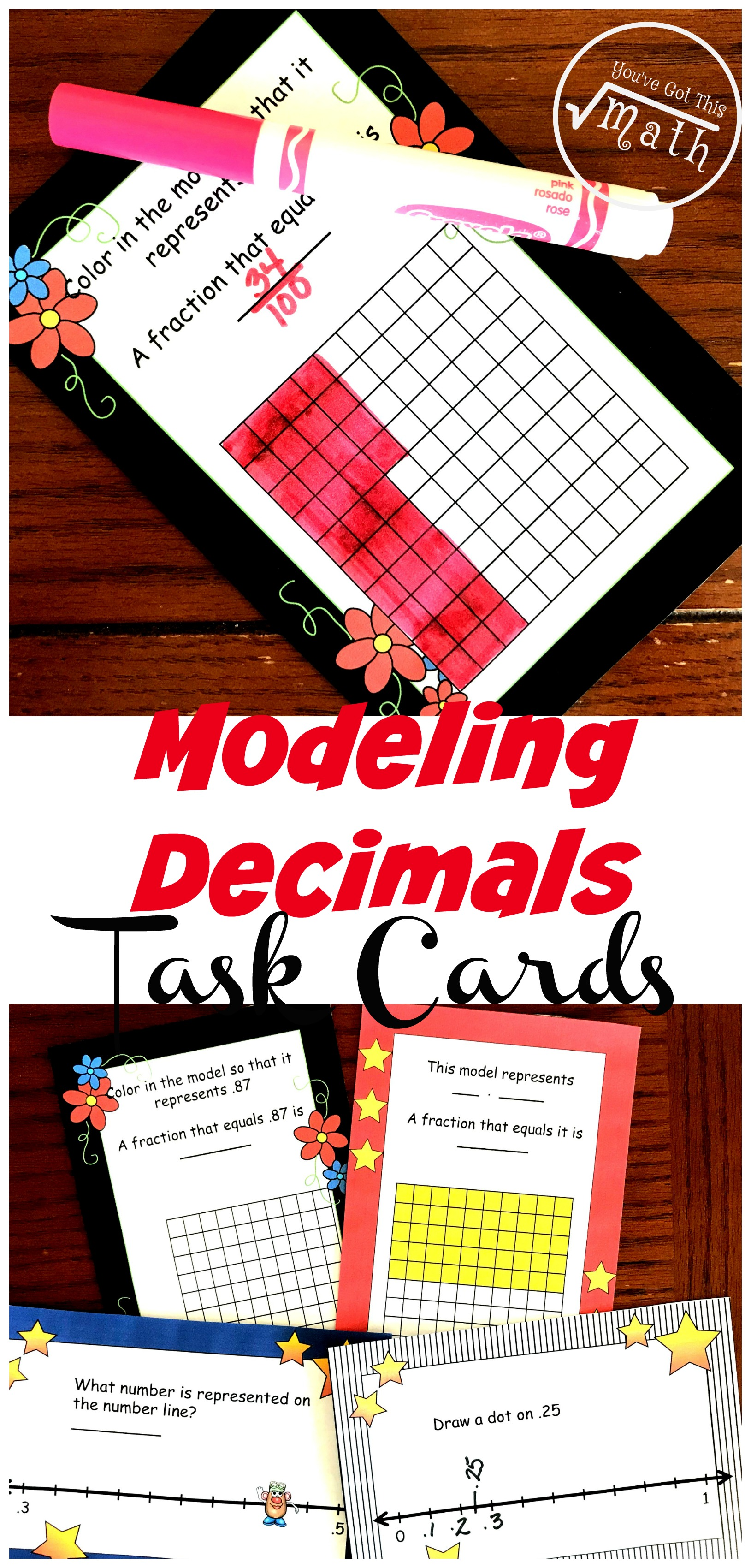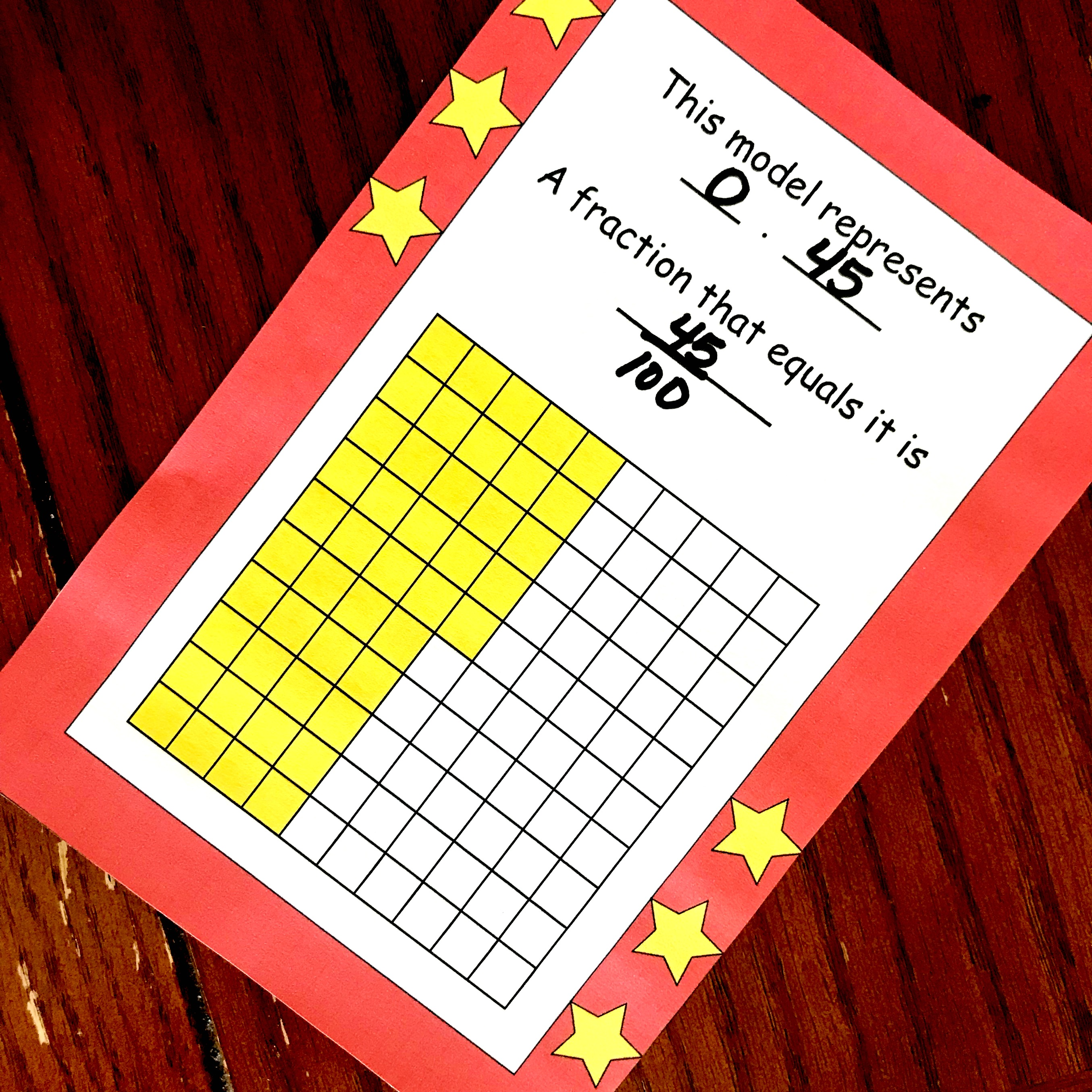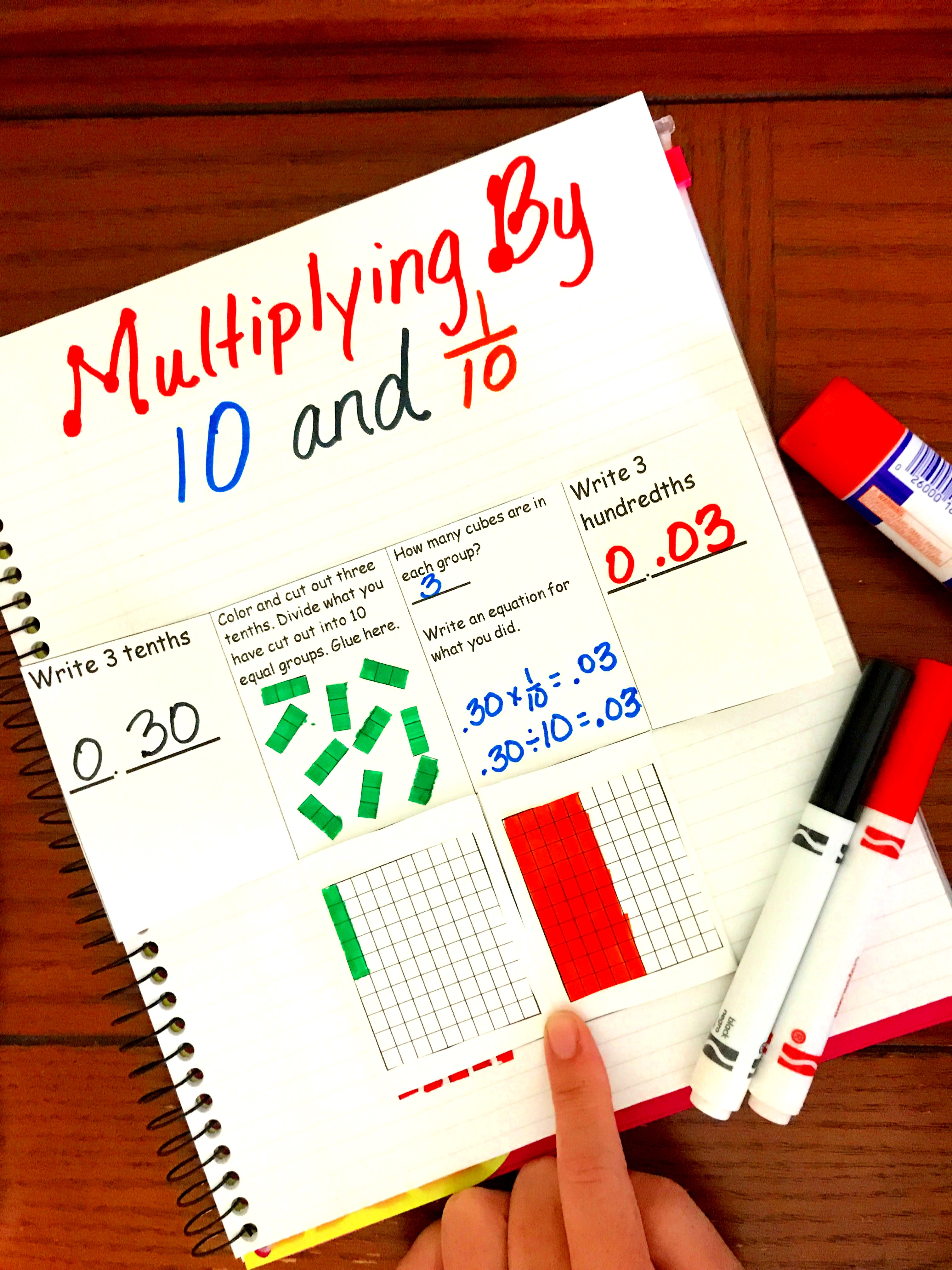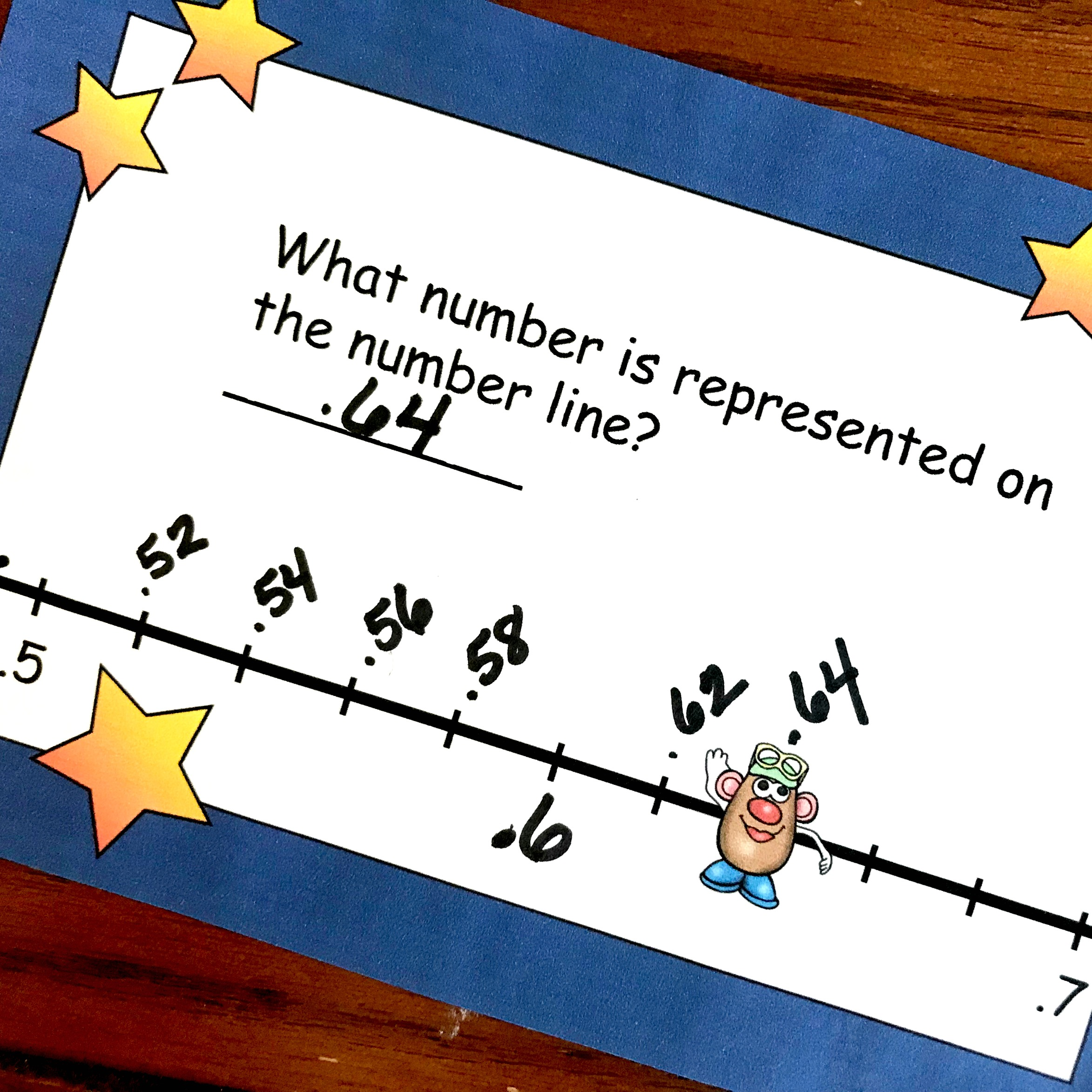Home » Math » 16 Task Cards To Practice Modeling Decimals on Number Lines and Decimal Grids

# 16 Task Cards To Practice Modeling Decimals on Number Lines and Decimal Grids

These task cards help children with modeling decimals. One set has children coloring a decimal grid to represent a decimal, while another has children figuring out the decimal represented on a decimal grid. The other two sets have children modeling decimals on number lines.Even if you have only read a few of my post, you have probably figured out how much I advocate modeling and exploring math concepts.

And today we are diving into modeling decimals.

## Prep-Work

To get ready to use this task cards, you have a couple of options.

First, print them off on card stock paper.

Then slide them into a sheet protector and provide dry earse markers.

Or……

Print them off on card stock paper.

Next, cut them out.

Finally, laminate and then provide dry erase markers.

## Decimal Grids:

#### Modeling Decimals: What is colored in?

Decimal grids are a fantastic way to help children relate decimals to fractions. If they are comfortable with the fact that a numerator is how many parts you have, and the denominator is what it takes to make a whole…..then it is easy to go from a fraction to a decimal.

Let’s look at this decimal grid.As you can see, there are 100 squares in the grid. So our denominator is 100. Forty-five squares are colored in so my numerator is 45. This grid represents 45/100.

To change this into a decimal, we must remember that value of the digits to the right of the decimal. The first value is the tenths while the second is the hundredths.

Let’s look at our model again. How many tenths are colored in? If you said four, you are correct.

So what is left on the decimal grid? Yes, five hundredths. The decimal represented in this grid is 0.45 and the fraction is 45/100.

Learn more about decimal place value with this interactive notebook.#### Modeling Decimals: Coloring in the Decimal Grid

What if you are given a decimal and asked to model it?

Well, let’s take a look at this one. The card asks for .34 to be colored in.Our first step is to look at the place value of each digit. The three is in the tenths spot, so we need to color in three tenths or three rows of the decimal grid.

Next, we look at the hundredths place. This tells us we need to color in four hundredths or four little squares.

Finally, we figure out the decimal. It is easy to see that 34 out of the hundred are colored in so my fraction is 34/100.

## Number Lines:

Modeling decimals can also be done on number lines!!

The trick is to figure out the intervals on the number line.In this example, you can see that the first number given is 0 and the last number given is 1. And it is important to note that there are 10 hopes between the 0 and 1. This means that the first blank line is 1 out of 10 hops, or 1/10 or 0.10.

If I’m going to label 0.25 like the card asks, I going to need to move up two-tenths or two hops. Then I have to focus on the five hundredths. Twenty-five hundredths is exactly in-between twenty-hundredths and thirty-hundredths, so my dot will be in between the second and third hop.

#### Number lines in the hundredths:This number line has different intervals. The first number we are given is five-tenths and the final number is seven-tenths. Once again there are 10 hops between the two numbers.

This requires a little more thought process but is very possible.

Let’s start by labeling the fifth hop or the line directly in the middle of five-tenths and seven-tenths. If you said, that six-tenths is in the middle, you are correct.

Now, we have to figure out the lines in-between. How can I get from five-tenths to six tenths in just five hops? Well, I definitely will be using hundredths. And I know that 20 x 5 = 100, so I’m going to count up by 2 hundredths. Once I label my whole number line, I can see that my potato head is located on 0.64!

As children work on modeling decimals using number lines and decimal grids their knowledge of decimal place value will grow.

Enjoy this time of learning and exploring with them.

You’ve Got This Rachel

You may also like:

Decimal Cut and Paste for Number Lines and Decimal GridsOr Get all my Decimals Printables in one place.### The free printable has 16 task cards, you can get all 40 here.